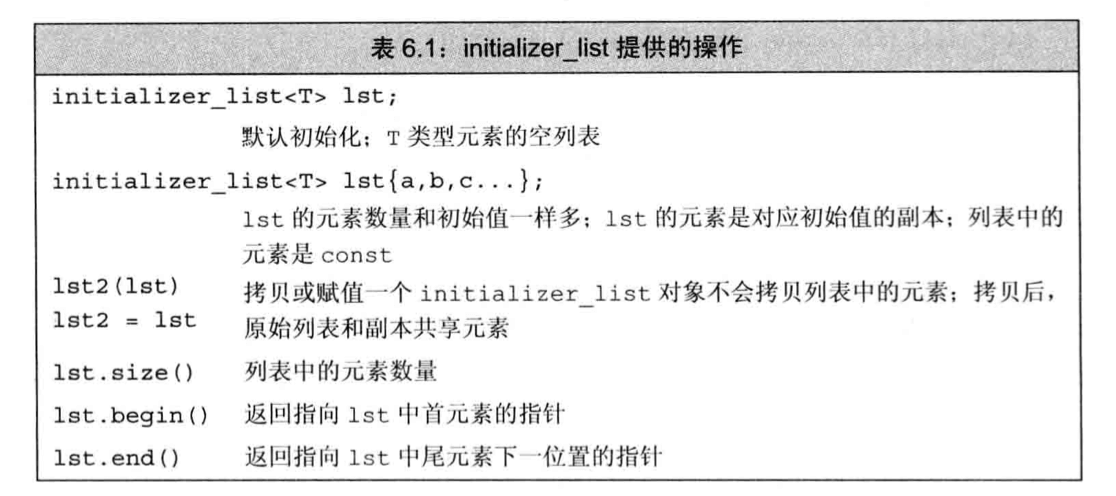# 【C++基础】第三十五课：参数传递

## 传值参数，传引用参数，const形参和实参，数组形参，main：处理命令行选项，含有可变形参的函数

Posted by x-jeff on December 29, 2021

【C++基础】系列博客为参考《C++ Primer中文版（第5版）》C++11标准）一书，自己所做的读书笔记。

# 2.传值参数

## 2.1.指针形参

1
2
3
4
5
6
7
8
9
10
11
12
13
14
15
16
17
18
19
20
21
22
23
24
void f(int *p, int *t, int *(&z)) {
int i = 100;
t = &i;
z = &i;
*p = 2;
cout << z << endl;//0x7ff7bdc31724
cout << *z << endl;//100
}

int main() {
int i = 42, v = 50;
int *p = &i;
int *t = &v;
int *z = &v;
cout << z << endl;//0x7ff7bdc317a4
f(p, t, z);
cout << i << endl;//2
cout << v << endl;//50
cout << *p << endl;//2
cout << *t << endl;//50
cout << *z << endl;//32759，这个需要额外注意！！！
cout << z << endl;//0x7ff7bdc31724
return 0;
}


# 3.传引用参数

1
2
3
4
5
6
7
8
9
void reset(int &i) {
i = 0;
}

int main() {
int j = 42;
reset(j);
cout << j << endl;//j=0
}


## 3.1.使用引用避免拷贝

1
2
3
4
5
//比较两个string对象的长度
bool isShorter(const string &s1, const string &s2)
{
return s1.size() < s2.size();
}


## 3.2.使用引用形参返回额外信息

1
2
3
4
5
6
7
8
9
10
11
12
13
14
15
string::size_type find_char(const string &s, char c, string::size_type &occurs)
{
auto ret=s.size();
occurs=0;
for(decltype(ret) i=0;i!=s.size();++i)
{
if(s[i]==c)
{
if(ret==s.size())
ret=i;
++occurs;
}
}
return ret;
}


# 4.const形参和实参

1
2
3
4
void fcn(const int i)
{
/*fcn能够读取i，但是不能向i写值*/
}


1
2
void fcn(const int i) { /*...*/ }
void fcn(int) { /*...*/ } //错误：重复定义了fcn(int)


## 4.1.尽量使用常量引用

1
string::size_type find_char(string &s, char c, string::size_type &occurs);


1
find_char("Hello World",'o',ctr);


# 5.数组形参

1
2
3
4
//尽管形式不同，但这三个print函数是等价的
void print(const int*);
void print(const int[]);
void print(const int);//这里的维度表示我们期望数组含有多少元素，实际不一定


1
2
3
int i=0,j={0,1};
print(&i);//正确：&i的类型是int*
print(j);//正确：j转换成int*并指向j


## 5.1.使用标记指定数组长度

1
2
3
4
5
6
void print(const char *cp)
{
if(cp) //若cp不是一个空指针
while(*cp) //只要指针所指的字符不是空字符
cout<<*cp++; //输出当前字符并将指针向前移动一个位置
}


## 5.2.使用标准库规范

1
2
3
4
5
void print(const int *beg, const int *end)
{
while(beg!=end)
cout<<*beg++<<endl;
}


## 5.3.显式传递一个表示数组大小的形参

1
2
3
4
5
6
7
void print(const int ia[], size_t size)
{
for(size_t i=0;i!=size;++i)
{
cout<<ia[i]<<endl;
}
}


1
2
int j[]={0,1};
print(j,end(j)-begin(j));


## 5.4.数组引用形参

C++语言允许将变量定义成数组的引用，基于同样的道理，形参也可以是数组的引用：

1
2
3
4
5
void print(int (&arr))
{
for(auto elem : arr)
cout<<elem<<endl;
}


⚠️&arr两端的括号必不可少：

1
2
f(int &arr) //错误：将arr声明成了引用的数组
f(int (&arr)) //正确：arr是具有10个整数的整型数组的引用


## 5.5.传递多维数组

1
2
3
4
5
//matrix指向数组的首元素，该数组的元素是由10个整数构成的数组
void print(int (*matrix), int rowSize)
{
/*...*/
}


⚠️*matrix两端的括号必不可少：

1
2
int *matrix; //10个指针构成的数组
int (*matrix); //指向含有10个整数的数组的指针


1
2
3
4
5
//等价定义
void print(int matrix[], int rowSize)
{
/*...*/
}


matrix的声明看起来是一个二维数组，实际上形参是指向含有10个整数的数组的指针。

# 6.main：处理命令行选项

1
int main() {...}


1
int main(int argc, char *argv[]) {...}


1
int main(int argc, char **argv) {...}

• argc是argument count的缩写。
• argv是argument vector的缩写。

1
prog -d -o ofile data0


1
2
3
4
5
6
argv="prog";//或者argv也可以指向一个空字符串
argv="-d";
argv="-o";
argv="ofile";
argv="data0";
argv=0;//最后一个指针之后的元素值保证为0


⚠️当使用argv中的实参时，一定要记得可选的实参从argv开始；argv保存程序的名字（路径），而非用户输入。

# 7.含有可变形参的函数

C++还有一种特殊的形参类型（即省略符），可以用它传递可变数量的实参。不过需要注意的是，这种功能一般只用于与C函数交互的接口程序。

## 7.1.initializer_list形参1
2
initializer_list<string> ls;
initializer_list<int> li;


⚠️和vector不一样的是，initializer_list对象中的元素永远是常量值，我们无法改变initializer_list对象中元素的值。

1
2
3
4
5
6
void error_msg(initializer_list<string> il)
{
for(auto beg=il.begin();beg!=il.end();++beg)
cout<<*beg<<" ";
cout<<endl;
}


1
2
3
4
5
//expected和actual是string对象
if (expected != actual)
error_msg({"functionX",expected,actual});
else
error_msg({"functionX","okay"});


1
2
3
4
5
6
7
void error_msg(ErrCode e, initializer_list<string> il)
{
cout<<e.msg()<<": ";
for(const auto &elem : il)
cout<<elem<<" ";
cout<<endl;
}


## 7.2.省略符形参

⚠️参略符形参应该仅仅用于C和C++通用的类型。特别应该注意的是，大多数类类型的对象在传递给省略符形参时都无法正确拷贝。

1
2
void foo(parm_list, ...);
void foo(...);


1
2
3
4
5
6
7
8
9
10
11
12
13
14
15
16
17
18
19
20
21
#include <stdio.h>
#include <stdarg.h>

void ArgFunc(const char *str, ...) {
va_list ap;
int n = 3;
char *s = NULL;
int d = 0;
double f = 0.0;
va_start(ap, str);
s = va_arg(ap, char*);
d = va_arg(ap, int);
f = va_arg(ap, double);//浮点最好用double类型，而不要用float类型；否则数据会有问题
va_end(ap);
printf("%s is %s %d, %f", str, s, d, f);//The answer is Hello 345, 788.234000
}

int main() {